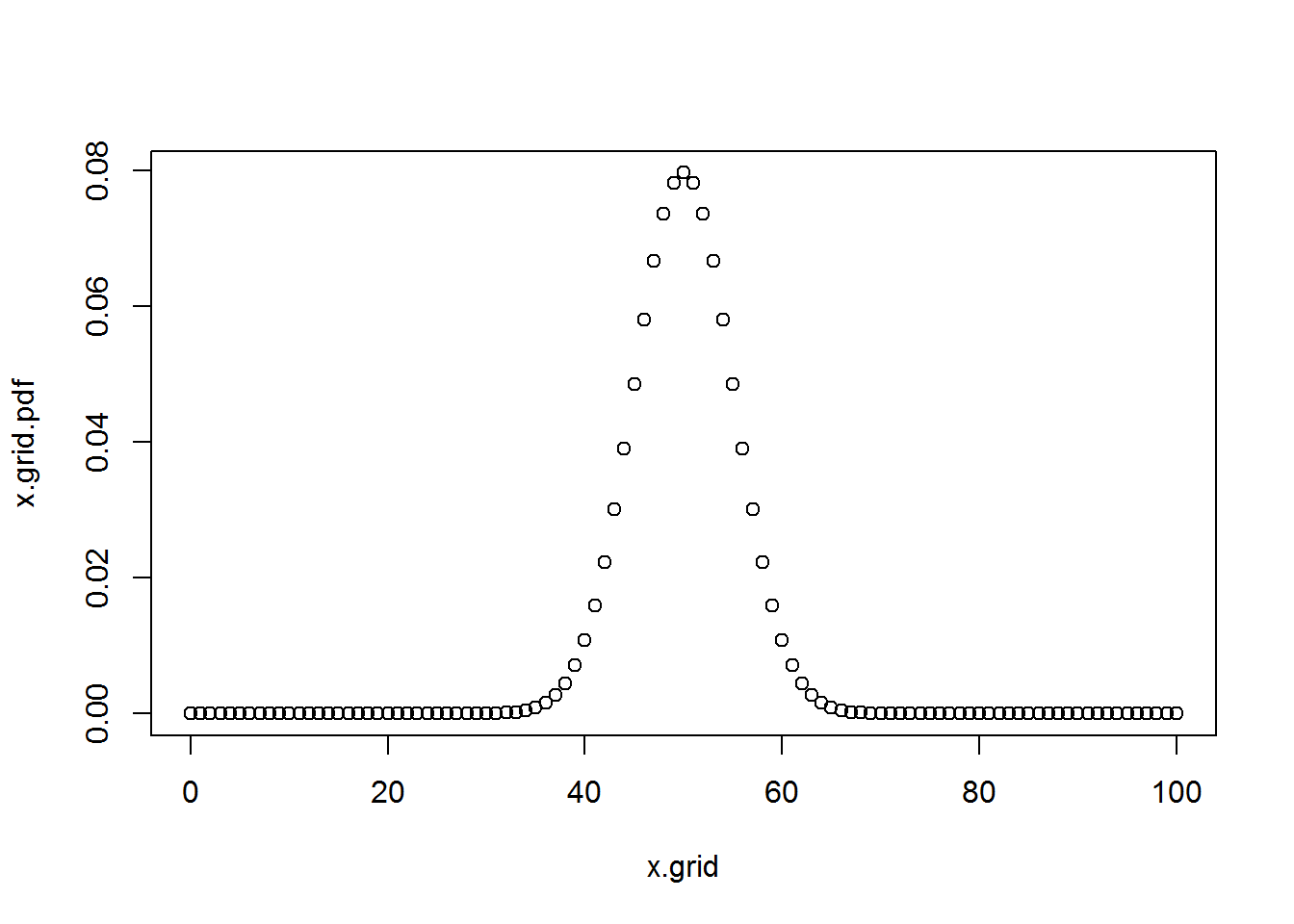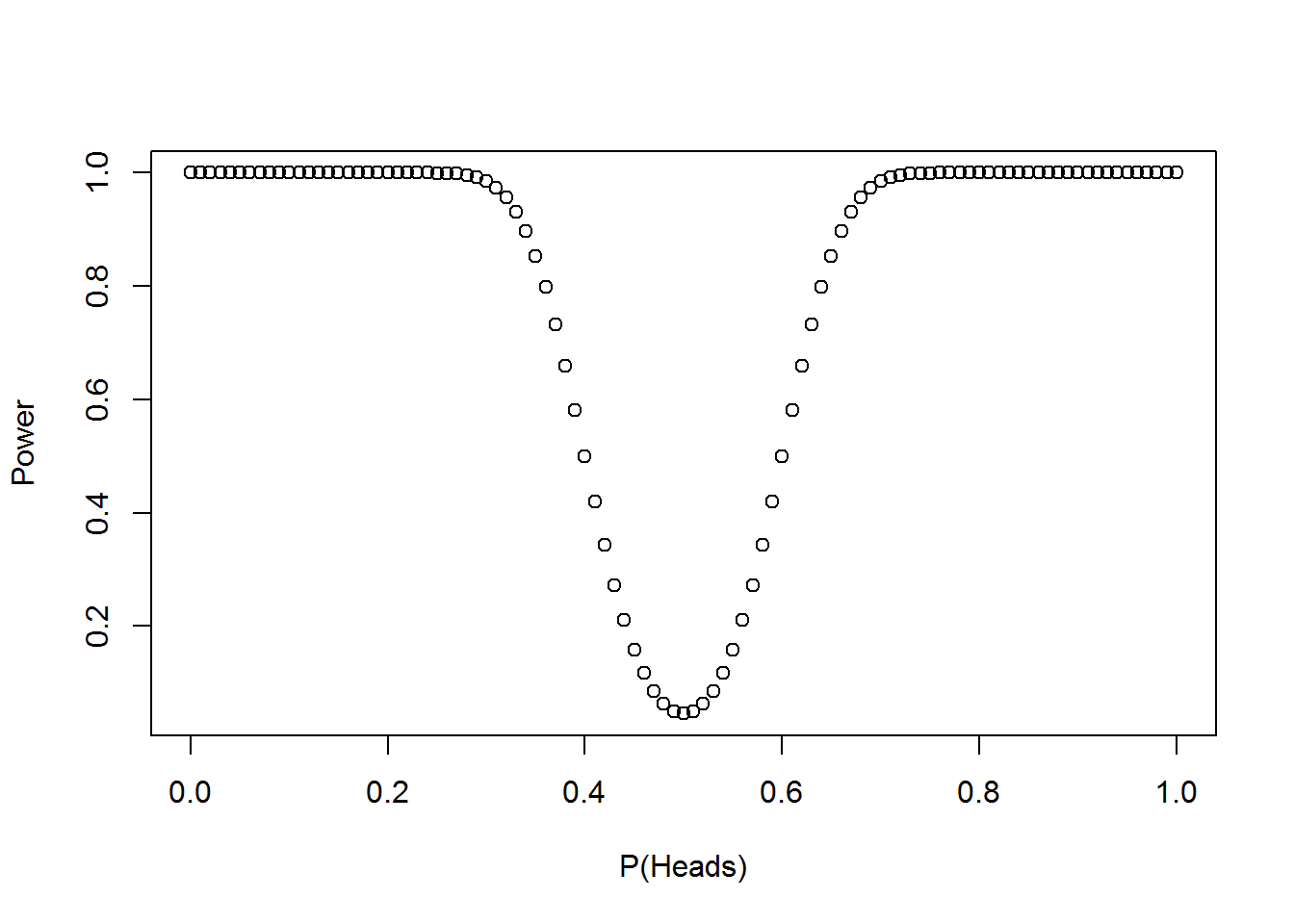# Problem 9.11.3

# X is a binomial(size=100,prob=p) random variable.

# Plot of the probability mass function of X for p=.5
x.grid=seq(0,100,1)
x.grid.pdf=dbinom(x.grid,size=100,prob=.5)
plot(x.grid, x.grid.pdf)# Consider the test of Null: p=0.5 vs Alternative p not =.5
#   that rejects when abs(x-50)>10
#
# The normal approximation for X is
#   Normal with mean = 100*p and Variance = 100*p*(1-p).

# a). What is alpha, the level of the test?

#   The test rejects when
#       abs(x-50) > 10
#   The standarized x value  is
#         z=(x-50)/sigma.x
#   Under the null distribution
#         sigma.x=sqrt(100*p*(1-p))=sqrt(100*.5*.5)=5
#   So, the test rejects when
#         abs(z) > 10/5 =2
#   and the level of the test is this probability, 0.0455.
sigma.x=sqrt(100*.5*(1-.5))

test.level=2*(1-pnorm(10/sigma.x))

# b). Graph the power as a function of p

grid.p=seq(0,1,.01)
# For each case of p, compute the rejection points of the
# standardized x value
# z.rejectlow=(40-100*p)/sqrt(p*(1-p)*100)
# z.rejecthigh=(60-100*p)/sqrt(p*(1-p)*100)

z.rejectlow=(40-100*grid.p)/sqrt(grid.p*(1-grid.p)*100)
z.rejecthigh=(60-100*grid.p)/sqrt(grid.p*(1-grid.p)*100)

grid.p.power= pnorm(z.rejectlow) + 1-pnorm(z.rejecthigh)

plot(grid.p, grid.p.power,xlab="P(Heads)",ylab="Power")# Note that the alpha (significance level) of the test
# is the value of the power for the null hypothesis p=.5

min(grid.p.power)
##  0.04550026
grid.p[which(grid.p.power==min(grid.p.power))]
##  0.5